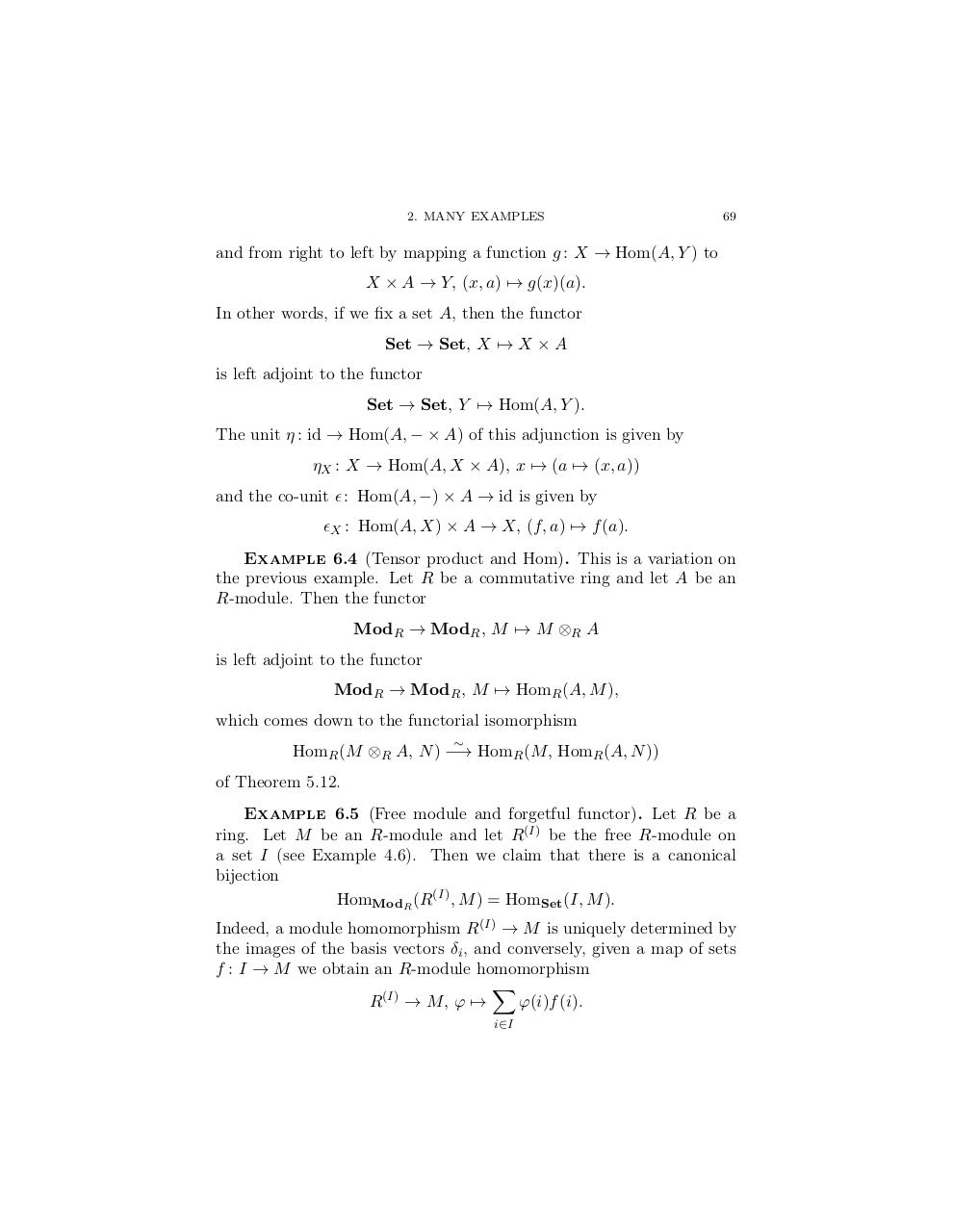# yoneda lenny.pdfPage 1 2 3 4 5 6 7 8 9

#### Text preview

2. MANY EXAMPLES

69

and from right to left by mapping a function g : X → Hom(A, Y ) to
X × A → Y, (x, a) 7→ g(x)(a).
In other words, if we fix a set A, then the functor
Set → Set, X 7→ X × A
is left adjoint to the functor
Set → Set, Y 7→ Hom(A, Y ).
The unit η : id → Hom(A, − × A) of this adjunction is given by
ηX : X → Hom(A, X × A), x 7→ (a 7→ (x, a))
and the co-unit  : Hom(A, −) × A → id is given by
X : Hom(A, X) × A → X, (f, a) 7→ f (a).
Example 6.4 (Tensor product and Hom). This is a variation on
the previous example. Let R be a commutative ring and let A be an
R-module. Then the functor
ModR → ModR , M 7→ M ⊗R A
is left adjoint to the functor
ModR → ModR , M 7→ HomR (A, M ),
which comes down to the functorial isomorphism

HomR (M ⊗R A, N ) −→ HomR (M, HomR (A, N ))
of Theorem 5.12.
Example 6.5 (Free module and forgetful functor). Let R be a
ring. Let M be an R-module and let R(I) be the free R-module on
a set I (see Example 4.6). Then we claim that there is a canonical
bijection
HomModR (R(I) , M ) = HomSet (I, M ).
Indeed, a module homomorphism R(I) → M is uniquely determined by
the images of the basis vectors δi , and conversely, given a map of sets
f : I → M we obtain an R-module homomorphism
X
R(I) → M, ϕ 7→
ϕ(i)f (i).
i∈I